Advances in Wireless Communications and Networks
Volume 2, Issue 2, November 2016, Pages: 15-24

USRP Implementation of PTS Technique for PAPR Reduction in OFDM Using LABVIEW

Mamdouh Elsayed Gouda1, Mohamed Hussien Moharam2, Mohamed Rabie Ragab2, Ahmed Mahmoud. Anwar2, Ahmed Fathi Gouda2

1Electronics and Communications Engineering Department, ElGazeera High Institute for Computer & Management Information, Cairo, Egypt

2Electronics and Communications Engineering Department, Misr University for Science and Technology (MUST), Giza, Egypt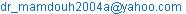(M. E. Gouda)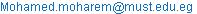(M. H. Moharam)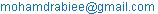(M. R. Ragab)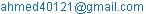(A. Mahmoud. Anwar)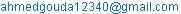(A. F. Gouda)

Mamdouh Elsayed Gouda, Mohamed Hussien Moharam, Mohamed Rabie Ragab, Ahmed Mahmoud. Anwar, Ahmed Fathi Gouda. USRP Implementation of PTS Technique for PAPR Reduction in OFDM Using LABVIEW. Advances in Wireless Communications and Networks. Vol. 2, No. 2, 2016, pp. 15-24. doi: 10.11648/j.awcn.20160202.11

Received: April 26, 2016; Accepted: June 22, 2016; Published: January 10, 2017

Abstract: Orthogonal frequency-division multiplexing (OFDM) is an orthogonal waveform technique for encoding digital data on multiple carrier frequencies. OFDM has developed into a popular scheme for wide band digital communication, used in applications such as wireless networks and 4G mobile communications such as LTE, WIMAX and Wifi. It still exists with some of the drawbacks, out of which, high peak to average power ratio (PAPR) gives rise to non-linear distortion, inter-symbol interference and out-of-band radiation. There has been various ways developed and implemented to reduce PAPR. we will describe all of these techniques to reduce PAPR, then focus on technique called Partial Transmit Sequence technique(PTS) which comes under Signal scrambling techniques. In this technique, the symbol in the frequency domain is partitioned into smaller disjoint sub-blocks. The objective is to design an optimal phase for the sub-block set that minimizes the PAPR. In this paper, an efficient Universal Software Radio Peripheral (USRP) implementation of PTS scheme for PAPR reduction OFDM signals using LABVIEW has been carried out.

Keywords: OFDM, PAPR, CCDF, PTS, Reduction Techniques, USRP, LABVIEW, National Instruments

Contents

1. Introduction

Orthogonal Frequency Division Multiplexing (OFDM) is an orthogonal multicarrier communication system. Bandwidth efficiency, high data rate and immune to fading makes the OFDM systems preferred choice for modern communication system and are being implemented in many modern communication systems  like Digital Audio Broadcasting (DAB), Digital Video Broadcasting (DVB), Wireless Local Area Network (WLAN) and Long Term Evolution (LTE) . Although OFDM gives many advantages, it suffers from many technical difficulties. Few of these difficulties include tight frequency synchronization, time offset, peak to average power ratio (PAPR) and channel estimation. In a multicarrier communication system, like OFDM, independent phases of subcarriers may have constructive or destructive effect. When all subcarriers have same phase then the constructive effect gives high peak amplitude and produces high peak to average power ratio (PAPR). The high PAPR drives the power amplifier to operate in non-linear region which causes inter-modulation distortions and out-of-band radiations. So, it is highly essential to reduce PAPR. For the same, various techniques have been employed such as coding, companding, amplitude clipping and filtering, active constellation extension (ACE), tone reservation (TR), tone injection (TI), selected mapping (SLM), partial transmit sequence (PTS). These schemes can mainly be categorized into signal scrambling techniques, such as PTS, and signal distortion techniques such as clipping, companding techniques. Among these methods, PTS scheme is the most efficient approach and a distortionless scheme for PAPR reduction by optimally combining signal subblocks .

In PTS technique, the input data block is broken up into disjoint sub-blocks. The sub-blocks are multiplied by phase weighting factors and then added together to produce alternative transmit containing the same information. The phase weighting factors, whose amplitude is usually set to 1, are selected such that the resulting PAPR is minimized. The number of allowed phase factors should not be excessively high, to keep the number of required side information bits and the search complexity within a reasonable limit. However, the exhaustive search complexity of the PTS technique increases exponentially with number of sub-blocks, so it is practically not realizable for a large number of sub-blocks. To find out a best weighting factor is a complex and difficult problem . In this paper, PTS implements on USRP using LABVIEW.

The paper is organized as follows. Section II briefly shows the OFDM signal model. In Section III the PAPR problem of the OFDM system is presented. In Section V The analysis of the PTS technique are described. In Section VI Simulation results using LABVIEW are provided. In Section VII Implementation results using USRP are provided. Finally conclusions are drawn in Section VIII.

2. OFDM System Model

In an OFDM system, the serial data stream to be transmitted is divided into parallel data stream constituting series of frames. All bits/symbols in a frame is modulated by,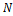subcarriers,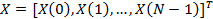Which are orthogonal. This is achieved by considering,

∆f=Where,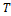denotes the duration of OFDM symbol, ∆f is the subcarrier spacing and,is number of subcarriers. After modulation, the frequency domain symbol is converted to time domain symbol with an,-point IFFT operation. The transmitted symbol is given by,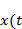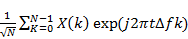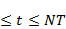(1)

Where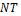is the data block period.

The basic building blocks of OFDM transceiver are the FFT and IFFT blocks. At the transmitter side IFFT is implemented whereas at the receiver side FFT is implemented. At OFDM Transmitter: The incoming serial data stream is converted to parallel blocks of data, with the number of elements in one parallel block being equal to the number of sub-carriers, say N. The parallel block of data is then passed through an N-point IFFT block to obtain the OFDM symbol. Thus the OFDM symbol is in digital time domain. At OFDM Receiver: OFDM signal is passed through a channel to the receiver which is then converted from serial to parallel stream. This parallel stream of OFDM signal data is de-mapped or demodulated by any of the demodulation technique BPSK or QPSK. This demodulated data is transformed from time domain to frequency domain with the operation of Fast Fourier Transform (FFT). Finally, the frequency domain signal is converted from parallel to serial and is received at the receiver .

The Block diagram of OFDM System is illustrated in the following Figure 1.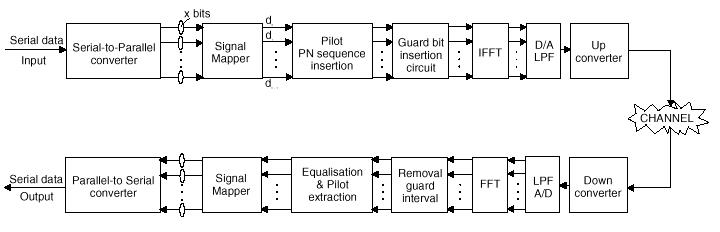Figure 1. Block diagram of OFDM System.

3. Peak to Average Power Ratio

High Peak-to-Average Power Ratio (PAPR) has been recognized as one of the major practical problem involving OFDM modulation. High PAPR results from the nature of the modulation itself where multiple subcarriers/sinusoids are added together to form the signal to be transmitted. When N sinusoids add, the peak magnitude would have a value of N, where the (rms) average might be quitelow due to destructive interference between the sinusoids. High signals are usually undesirable for it usually strains the analog circuitry. High PAPR signals would require a large range of dynamic linearity from the analog circuits, which usually results in expensive devices, and higher power consumption/lower efficiency (for example, power amplifier has to operate with larger backoff to maintain linearity) . It’s quite straightforward to observe that in OFDM systems, some input sequences would result in higher PAPR than others. Theoretically, large peaks in OFDM system can be expressed as PAPR. It is defined as: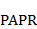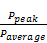(2)

Where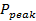represents peak output power,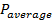represents means average output power and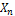represents the transmitted OFDM signals.

Mathematically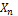is expressed as: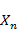(3)

Where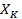represents the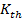input symbol.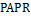is generally represented by a complementary cumulative distribution function (CCDF) where x-axis denotes the preset threshold and y-axis denotes the probability that the PAPR exceeds this threshold.

The CCDF is defined by,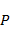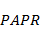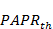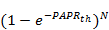(4)

Where,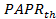is the threshold  .

One of the major disadvantages of OFDM systems is that the OFDM signal has high (PAPR), and to deal with this problem many typical techniques have been proposed. Each technique is different from the other in its complexity and performance. Thereduction techniques are listed in Table 1.

Table 1. Classification of PAPR Reduction Techniques.

 Reduction Techniques  Signal Scrambling Techniques Signal Distortion Techniques With Explicit Side Information Without Explicit Side Information Signal Clipping Coding based Probabilistic Schemes Hadamard Transform Method Peak Windowing Block Coding Schemes Selective Level Mapping Dummy Sequence Insertion Envelope Scaling Sub Block Coding Schemes Partial Transmit Sequence Random Phase Updating Block Coding With Error Correction Interleaving Peak Reduction Carrier Tone reservation Companding Tone injection Active Constellation Extension Linear Block Coding

4. Partial Transmit Sequence (PTS)

In PTS, the original data block is divided into multiple non-overlapping sub-blocks. Then these sub-blocks are rotated with rotation factors which are statistically independent. After that, the signal with the lowest PAPR is chosen for transmission. There are several ways for the partition of the data sequence into multiple sub-blocks, including adjacent partition, interleaved partition and pseudorandom partition. The major drawback of PTS is also the computational complexity (search complexity for optimal phase factor, and more than one IFFT blocks) and low data rate (required side information). Several techniques have been

proposed in the literature to reduce the search complexity and overhead (by reducing/avoiding the usage of side information). In PTS method, the original frequency-domain data sequence is divided into multiple disjoint sub-blocks, which are then weighted by a set of phase sequences to create a set of candidates finally, the candidate with the lowest PAPR is chosen for transmission . A block diagram of PTS techniques is shown in Figure 2 The input data block in X is divided in to V disjoint sub-blocks, which are represented by the vectors ,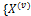,=0,1,…,V-1} The input data block X can be written in terms of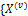} as

X=forV=0,1,…,V-1

Where,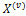=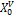,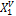,…,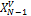with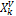=or 0

After that, the sub-blocks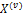are transformed into V, time-domain partial transmit sequences by taking the IFFT of length N. These partial transit sequences can be written as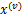=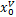,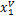,…,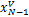=IFFT[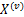] for V= 0,1,…, V – 1

These partial sequencesare then independently rotated by phase factors={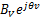}, for V = 0,1,…, V - 1 The rotated partial sequences are then optimally combined to obtain the OFDM signals with lowest PAPR. The time domain signal after combining is given by  :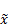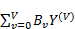(5)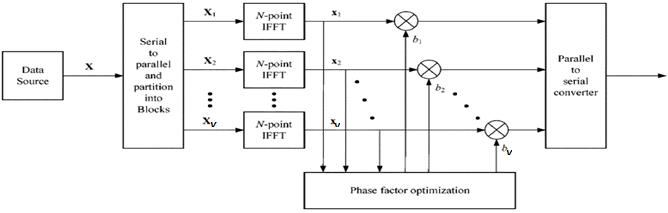Figure 2. TheBlock diagram of Partial Transmit Sequence (PTS) technique.

PTS Technique is distortion less due to which the BER performance is not affected in OFDM, can be implemented for large number of subcarriers In turn the complexity is not affected, provides flexibility to work with any number of subcarriers, is flexible for any type of modulation. It functions equally well with BPSK, QPSK, etc, is flexible for any type of modulation and any number of sub-blocks and the best method for peak to average power ratio reduction as compared to other techniques. But it also suffers from High computational complexity: Due to IFFT operation and the phase factor optimization this technique undergoes very high computational complexity . Suppose that there are phase angles to be allowed, thus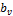can has the possibility of different values. Therefore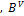alternative representations for an OFDM symbol. The search complexity increases exponentially with the number of sub-blocks V.

This is the major disadvantage of PTS technique but this technique is preferred only due to good PAPR reduction. Another disadvantage is the Loss in data rates: The side information is the main reason behind data rate loss. This takes place as because the phase factor rotation values needs to be send at receiver .

5. Simulation Results Using LabVIEW

LabVIEW is a graphical programming language developed by National Instruments. LabVIEW provides a simple inter-face for configuring and operating various external I/O, in-cluding the NI USRP hardware. Simulations were performed to compare the performance of PAPR reduction in OFDM symbols among OFDM without PTS and with PTS [9-11]. the parameter used for calculation of PAPR are illustrated in Table 2.

Table 2. The System Parameters Used for Simulation.

 Parameter Valued used Number of sub-carriers (N) 64 Oversampling factor (OF) 4 Modulation scheme 4,16,64-QAM Number of sub-blocks used in PTS methods (V) 4,16,32,64 Number of generated OFDM signal 10000 Number of phase factors (b) 4

Fig. 3 shows the CCDF of PAPR for a 4-QAM/OFDM system without PTS and with PTS technique as the number of subblock varies.Figure 3. CCDF of OFDM signals without PTS and with PTS of V=4, 16, 32, and 64 for4-QAM (using LABVIEW SIMULATION).

Fig. 4 shows the CCDF of PAPR for a 16-QAM/OFDM system without PTS and with PTS technique as the number of subblock varies.Figure 4. CCDF of OFDM signals without PTS and with PTS of V = 4, 16, 32, and 64 for 16-QAM (using LABVIEW SIMULATION).

Fig. 5 shows the CCDF of PAPR for a 64-QAM/OFDM system without PTS and with PTS technique as the number of subblock varies.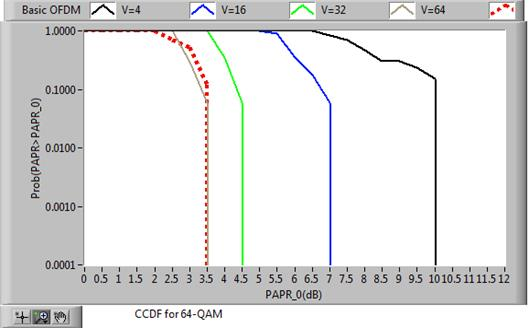Figure 5. CCDF of OFDM signals without PTS and with PTS of V = 4, 16, 32, and 64 for 64-QAM (using LABVIEW SIMULATION).

As shown in figure 3,4,5: It is seen that the PAPR performance improves as the number of sub blocks increases with V = 4, 16, 32, and 64. The Basic OFDM curve has PAPR equals to 10.5 dB. After applying PTS technique, the value was significantly reduced to 2.5 dB using V=64 for 4-QAM, 3 dB using V=64 for 16 QAM and 3.5 dB using V=64 for 64-QAM. This proves that PTS gives better results which is superior performance in PAPR reduction. It is seen that the PAPR performance improves as the number of sub blocks increases with V = 4, 16, 32, and 64. The CCDF results for simulation are illustrated in Table 3.

Table 3. The CCDF Results for Simulation.

 Modulation scheme Sub Block-partition- PAPR (dB) 4-QAM V=4 7 dB V=16 6 dB V=32 3.5 dB V=64 2.5 dB 16-QAM V=4 6 dB V=16 5 dB V=32 3.5 dB V=64 3 dB 64-QAM V=4 7 dB V=16 4.5 dB V=32 3.5 dB V=64 3.5 dB

6. Implementation Using USRP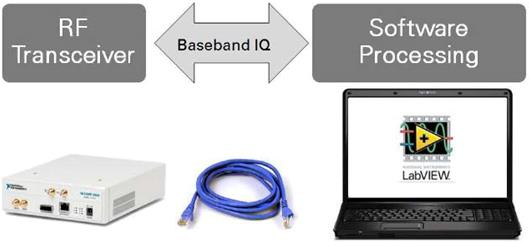Figure 6. Simplified Overview of a SDR Setup Built Around an NI USRP.

SDR refers to the technology wherein software modules running on a generic hardware platform are used to implement radio functions. Combine the NI USRP hardware with LabVIEW software for the flexibility and functionality to deliver a platform for rapid prototyping involving physical layer design, wireless signal record & playback, signal intelligence, algorithm validation, Implementation was performed to test simulations result through a real wireless communication link using two Universal Software Radio Peripheral (USRP 29-20) one as transmitter and the other as a receiver [9-12]. Figure 6 shows Simplified Overview of a SDR Setup Built around an NI USRP.

The parameters used for calculation of PAPR are illustrated in Table 4.

Table 4. The System Parameters Used for Implementation.

 Parameter Valued used Data Random bits Symbol rate 5 Msps Modulation schemes 4, 16, 64 QAM Number of subcarrier 64 Oversampling factor 4 Length of cyclic prefix 8 bit Number of sub-blocks used in PTS methods (V) 4, 16, 32, 64 Packet header/tail (8bit) IEEE 802.11a Short Training Channel encoding (3,1) repetition code Pulse shaping filter Raised cosine, α=0.5 TX average power level 0 dBm RX reference level -20 dBm

6.1. Implementation Results Using 4-QAM

Fig. 7 shows The PAPR characteristics of the PTS-OFDM signals which includes the distributions of the imaginary and real parts using various V for N=64 and 4-QAM.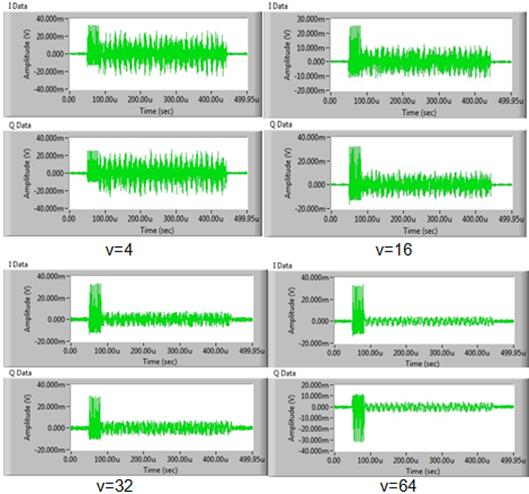Figure 7. Received signal for various V=4,16,32,64 using 4-QAM.

Fig. 8. Shows the PTS-OFDM signals at time domain using 4-QAM modulated subcarrier for N=64.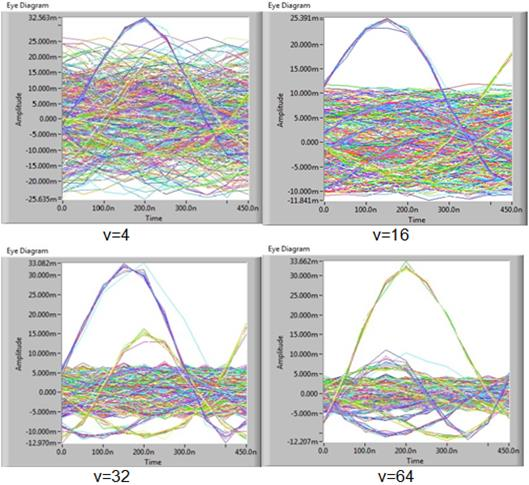Figure 8. Received signal in time domain for various V=4,16,32,64 using 4-QAM.

Fig. 9. shows the CCDF of PAPR for a 4-QAM/OFDM system without PTS and with PTS technique as the number of subblock varies.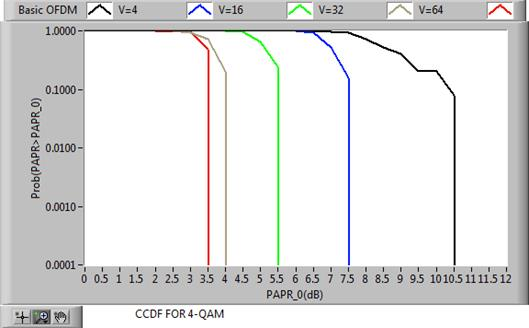Figure 9. CCDF of OFDM signals without PTS and with PTS of V = 4,16,32,and 64 for 4-QAM (using USRPs).

6.2. Implementation Results Using 16-QAM

Fig. 10 shows The PAPR characteristics of the PTS-OFDM signals which includes the distributions of the imaginary and real parts using various V for N=64 and 16-QAM.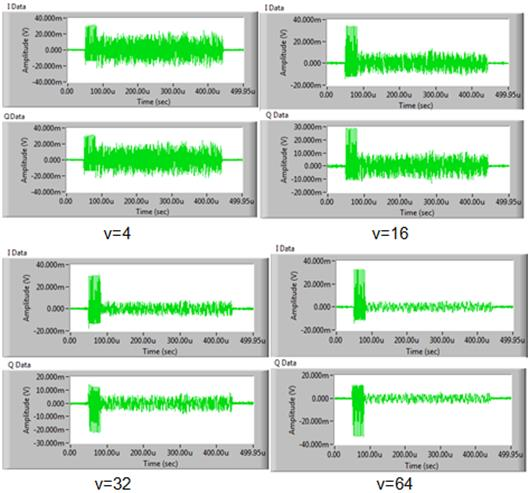Figure 10. Received signal for various V=4,16,32,64 using 16-QAM.

Fig. 11. shows the PTS-OFDM signals at time domain using 16-QAM modulated subcarrier for N=64.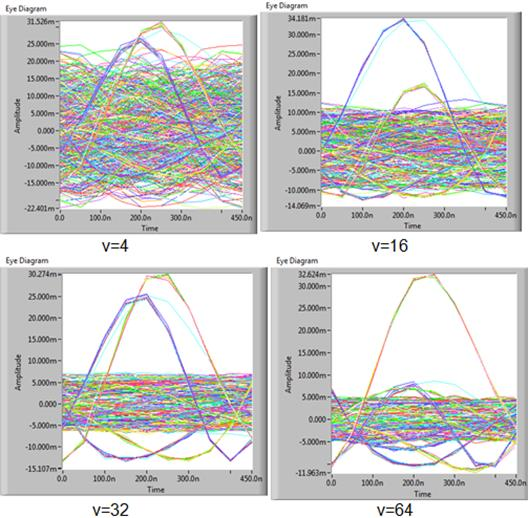Figure 11. Received signal in time domain for various V=4,16,32,64 using 16-QAM.

Fig. 12. shows the CCDF of PAPR for a 16-QAM/OFDM system without PTS and with PTS technique as the number of subblock varies.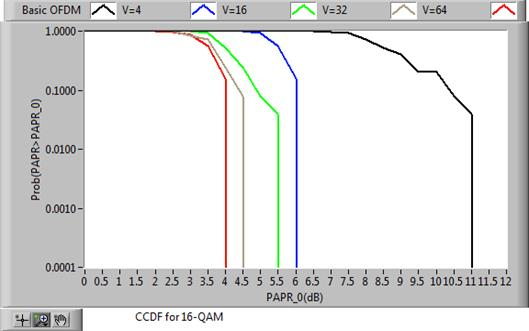Figure 12. CCDF of OFDM signals without PTS and with PTS of V = 4,16,32,and 64 for 16-QAM (using USRPs).

6.3. Implementation Results Using 64-QAM

Fig. 13 shows The PAPR characteristics of the PTS-OFDM signals which includes the distributions of the imaginary and real parts using various V for N=64 and 64-QAM.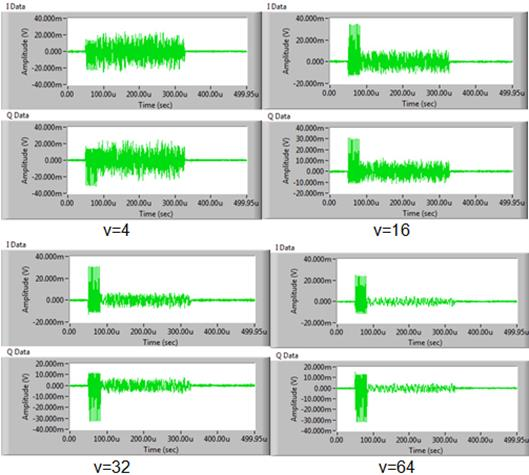Figure 13. Received signal for various V=4,16,32,64 using 64-QAM.

Fig. 14. shows the PTS-OFDM signals at time domain using 64-QAM modulated subcarrier for N=64.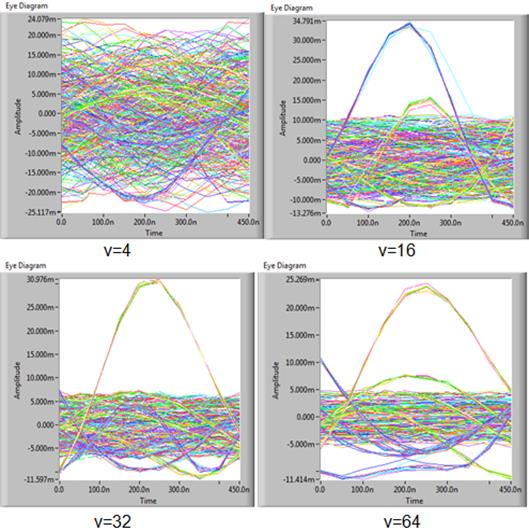Figure 14. Received signal in time domain for various V=4,16,32,64 using 64-QAM.

Fig. 15. shows the CCDF of PAPR for a 64-QAM/OFDM system without PTS and with PTS technique as the number of subblock varies.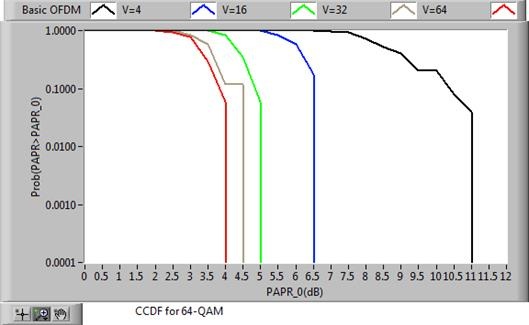Figure 15. CCDF of OFDM signals without PTS and with PTS of V = 4,16,32,and 64 for64-QAM (using USRPs).

The CCDF results for implementation are illustrated in Table 5.

Table 5. The CCDF Results for Implementation.

 Modulation scheme Sub Block-partition- PAPR (dB) 4-QAM V=4 7.5 dB V=16 5.5 dB V=32 4 dB V=64 3.5 dB 16-QAM V=4 6 dB V=16 5.5 dB V=32 4.5 dB V=64 4 dB 64-QAM V=4 6.5 dB V=16 5 dB V=32 4.5 dB V=64 4 dB

Implementation results show that there is 0.5 - 1.5 dB offset in CCDF curves among simulation and implementation due to USRP performance.

7. Conclusion

This paper provides an overview of Orthogonal-Frequency-Division-Multiplexing (OFDM). The purpose of this paper was to reduce the High (PAPR) of OFDM signals. The comprehensive research and comparison are put forward for a variety of currently promising PAPR reduction methods quoted in the literature in this research area. This paper presented a Partial transmit sequence method that was used to reduce the PAPR of OFDM signals and this was successfully achieved. Simulations and Implementations using LABVIEW and USRP were conducted and show that the performance of PTS method provides a good PAPR reduction.

References

1. Zainab Saad Hadi AL-Hashmi, An Overview: Peak to Average Power Ratio (PAPR) in OFDM system using some new PAPR techniques (with matlab code).Baghdad: Jordan, pp. 57-59, 2015.
2. Lim Dae-Woon, Heo Seok-Joong, No Jong-Seon, "An overview of peak-to-average power ratio reduction schemes for OFDM signals," IEEE Journal of Communications and Networks, DOI: 10.1109/JCN.2009.6391327, ISSN:1229-2370/7 2009.
3. Jeffery G. Andrews, Arunabha Ghosha, Rias Muhamed, Prentice Hall Fundamental of WIMAX Auston: Texes, pp.113-147, 2007.
4. Jyh-Horng Wen, Shu-Hong Lee, Yung-Fa Huang, Ho-Lung Hung. "A Suboptimal PTS Algorithm Based on Particle Swarm Optimization Technique for PAPR Reduction in OFDM Systems," EURASIP Journal on Wireless Communications and Networking, DOI: 10.1155/2008/601346, 25 June 2008.
5. Mamdouh Gouda, Mohamed Hussien. "Partial Transmit Sequence PAPR Reduction Method for LTE OFDM Systems," 2013 4th International IEEE Conference on Intelligent Systems, Modelling and Simulation, DOI 10.1109/ISMS.2013.78, ISSN 2166-0662/13 2013.
6. Seemanjali Sahoo,Sarat Kumar Patra, "VHDL Implementation of Circularly Shifted PTS Technique for PAPR Reduction in OFDM," 2014 International IEEE Conference on Advanced Communication Control and ComputingTechnologies (ICACCCT), DOI:10.1109/ICACCCT.2014.7019203, pp. 805 - 808/2014.
7. Mamdouh Gouda, Khaled Ali Shehata, Mohamed Hussien. "PAPR Reduction Performance for LTE OFDM Systems with Different Techniques" IJSER, ISSN 2229-5518, Volume 4, Issue 5, May 2013.
8. Renaldi Winoto, "Peak-to-Average Power Control in OFDM Systems," unpublished.
9. National Instruments, "Getting Started with LABVIEW", http://www.ni.com/pdf/manuals/373427j.pdf. 2013.
10. National Instruments, "NI USRP Hardware: Getting Started Guide", http://www.ni.com/pdf/manuals/375717e.pdf. 2013.
11. Robert W. Heath Jr., "Digital Wireless CommunicationPhysical Layer Exploration LabUsing the NI USRP". Auston: Texes, 2012 National Technology and Science Press.
12. National Instruments, "AN INTRODUCTION TO SOFTWARE DEFINED RADIO With NI LabVIEW and NI USRP" National Instruments issue 1.0 -2013 page.4

 Contents 1. 2. 3. 4. 5. 6. 6.1. 6.2. 6.3. 7.
Article ToolsAbstractPDF(4030K)